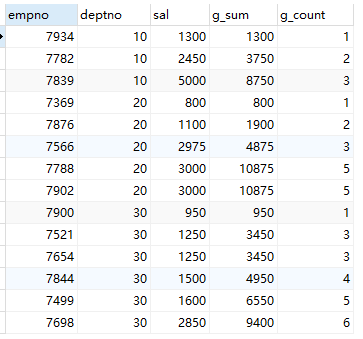## mysql聚合函数---总体聚合、总体累加、分组聚合、分组累加

MySQL从版本8.0开始，才支持窗口函数，所以之前的版本分组累加需要构造sql语句来实现。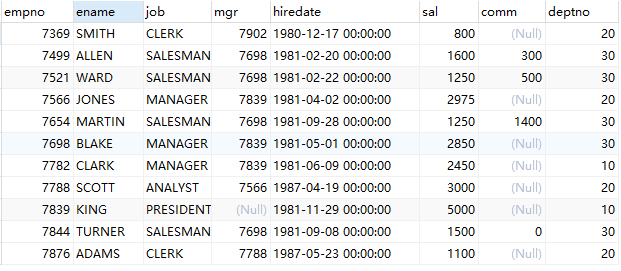# 一、mysql总体聚合函数

min()、max()、count()、sum()、avg()

``select count(ename) ,max(sal),min(sal),sum(sal),round(avg(sal),2) from emp;``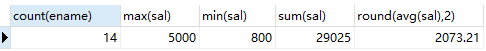# 二、mysql总体累加/总体累计数量

``````#累计求和/累计数量
select @s :=0 ,@rank := 0;
select *,@s := @s+sal , @rank := @rank+1 from emp order by sal;``````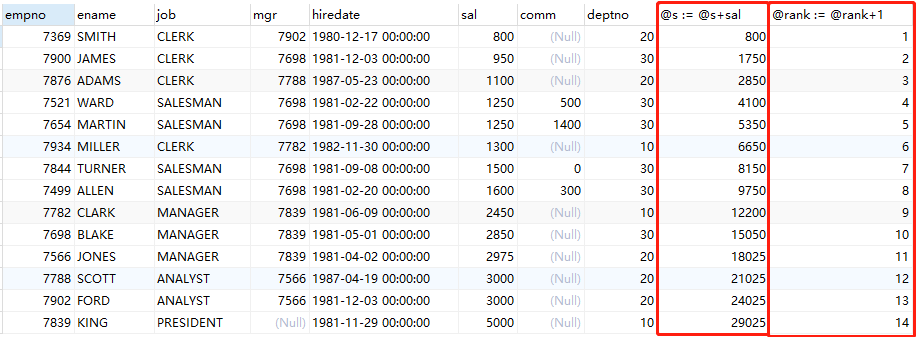# 三、分组聚合

``````select deptno,count(sal),sum(sal),round(avg(sal),2) from emp
group by deptno
order by avg(sal);``````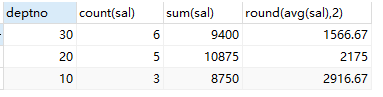``````select deptno,count(sal) from emp
group by deptno having deptno = 10 or deptno = 30   #只要10、30部门的数据
order by count(sal) desc;``````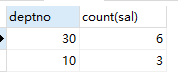``````select  deptno,count(sal) from emp  #计算各个部门sal>=2000的员工个数
where sal>=2000
group by deptno
order by count(sal) desc;``````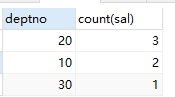# 四、分组累加/累计计数

``````select empno,deptno,sal ,
(select sum(sal) from emp where deptno = e.deptno and empno<=e.empno ) as g_sum
from emp as e order by deptno ,empno;``````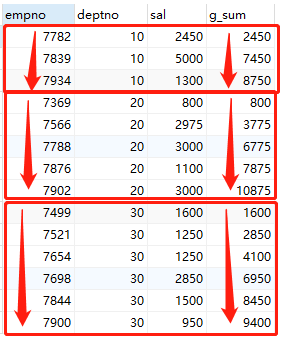``````select empno,deptno,sal ,
(select sum(sal) from emp where deptno = e.deptno and sal<=e.sal ) as g_sum,
(select count(sal) from emp where deptno = e.deptno and sal<=e.sal order by sal) as g_count
from emp as e order by deptno ,sal;``````